# An Introduction to corrplot package

## Introduction

The corrplot package is a graphical display of a correlation matrix, confidence interval.
It also contains some algorithms to do matrix reordering. In addition, corrplot is good at details, including choosing color, text labels, color labels, layout, etc.

## Visualization Methods

There are seven visualization methods (parameter method) in corrplot package, named "circle", "square", "ellipse", "number", "shade", "color", "pie".

data(mtcars)
M <- cor(mtcars)
corrplot(M, method = "circle")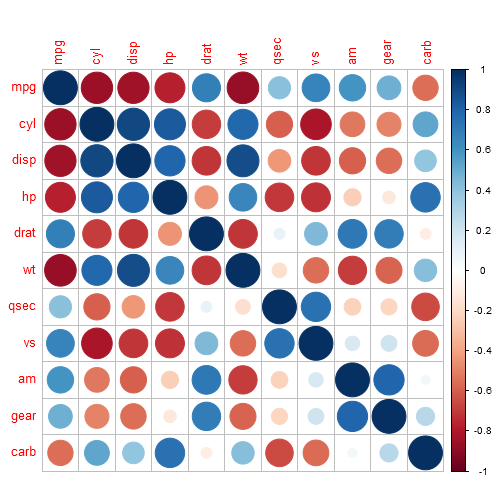corrplot(M, method = "square")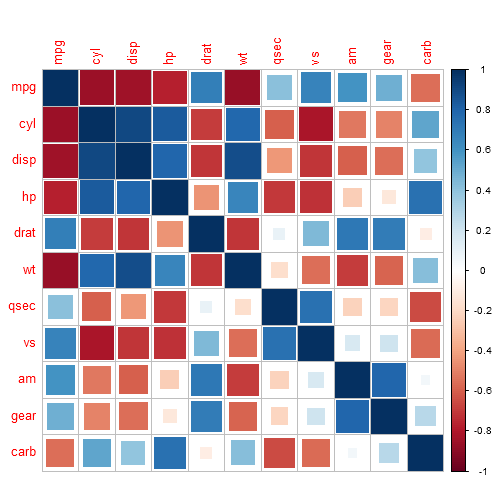corrplot(M, method = "ellipse")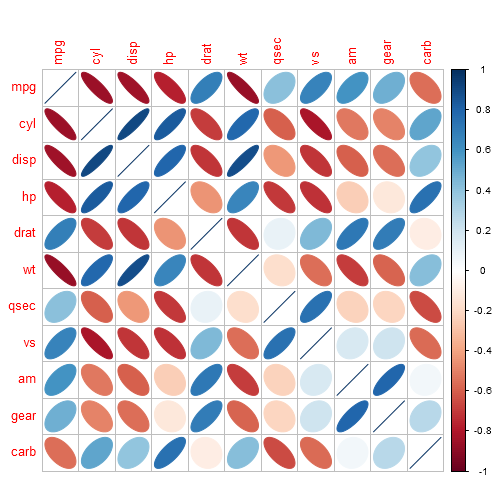corrplot(M, method = "number")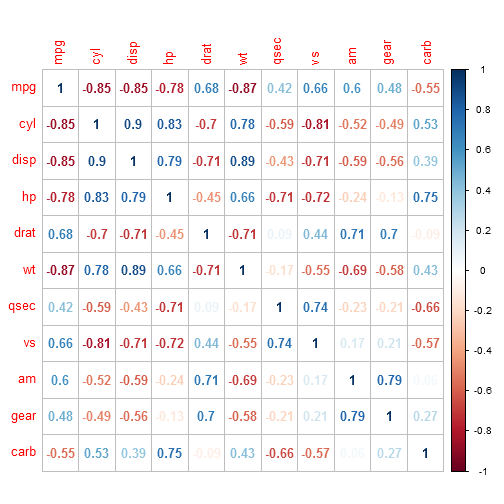corrplot(M, method = "shade")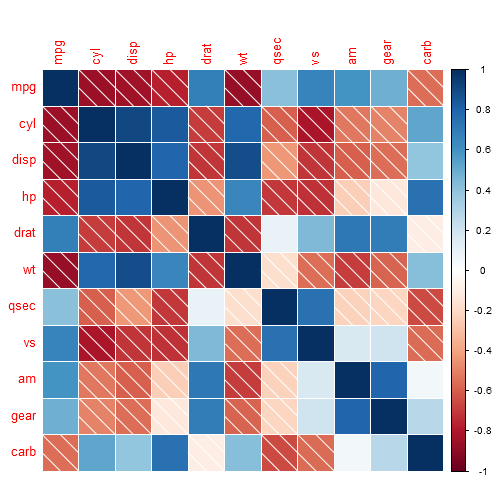corrplot(M, method = "color")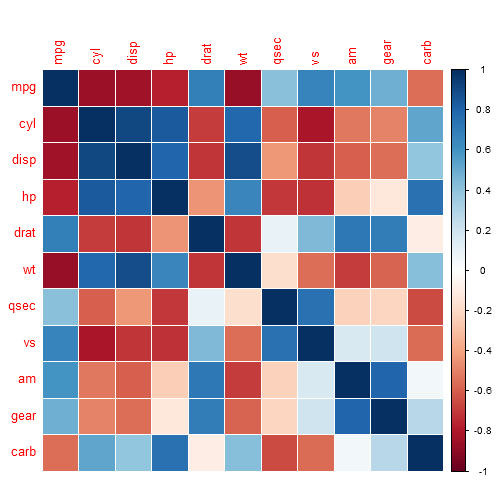corrplot(M, method = "pie")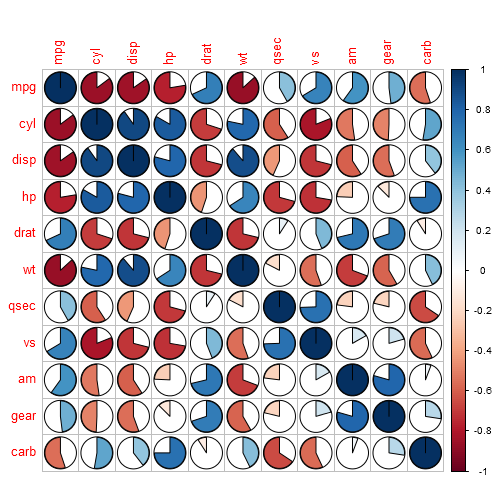## Layout

There are three layout types (parameter type), named "full" (default), "upper" or "lower", display full matrix, lower triangular or upper triangular matrix.

corrplot(M, type = "upper")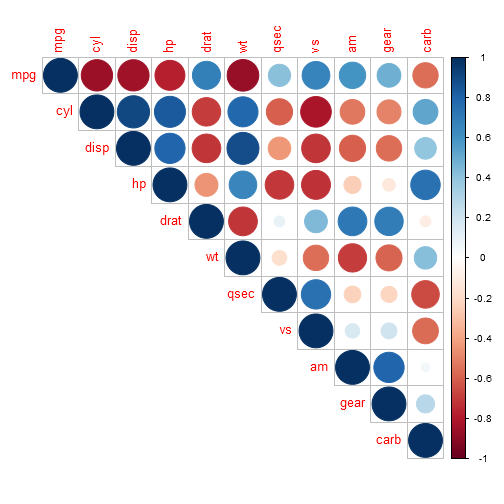corrplot(M, type = "lower")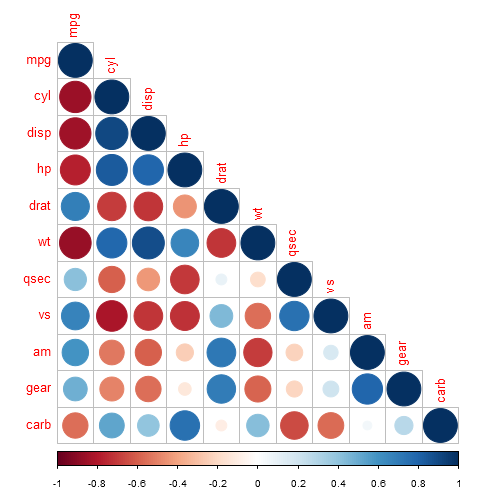corrplot.mixed() is a wrapped function for mixed visualization style.

corrplot.mixed(M)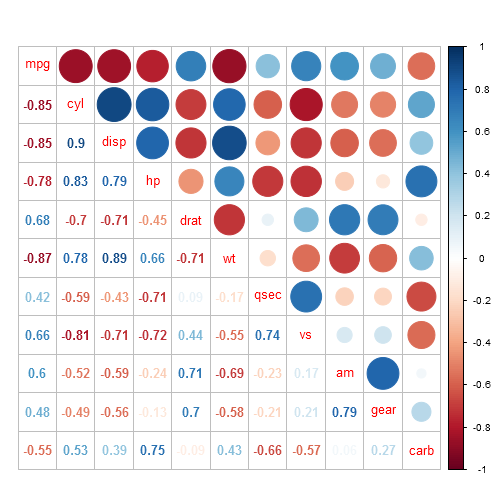corrplot.mixed(M, lower = "ellipse", upper = "circle")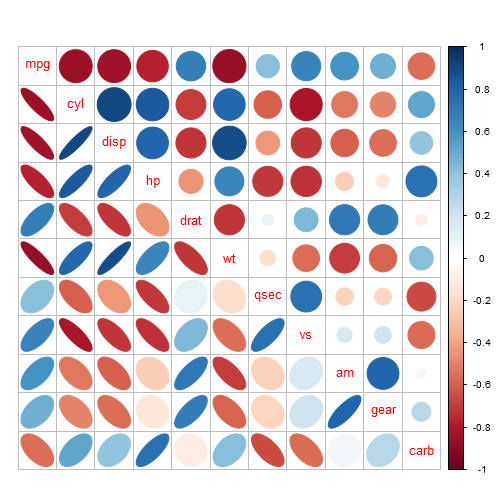corrplot.mixed(M, lower = "square", upper = "circle")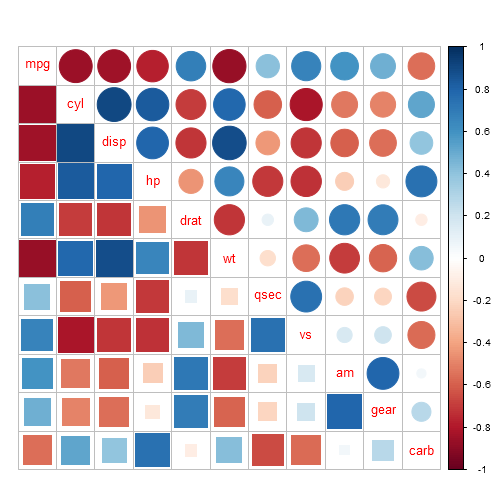## Reorder A Correlation Matrix

Matrix reorder is very important for mining the hiden structure and pattern in the matrix. There are four methods in corrplot (parameter order), named "AOE", "FPC", "hclust", "alphabet". More algorithms can be found in seriation package.

You can also reorder the matrix “manually” via function corrMatOrder().

• "AOE" is for the angular order of the eigenvectors. It is calculated from the order of the angles. $$a_i$$,

$a_i = \begin{cases} \tan (e_{i2}/e_{i1}), & \text{if e_{i1}>0;} \newline \tan (e_{i2}/e_{i1}) + \pi, & \text{otherwise.} \end{cases}$

where $$e_1$$ and $$e_2$$ are the largest two eigenvalues of the correlation matrix. See Michael Friendly (2002) for details.

• "FPC" for the first principal component order.

• "hclust" for hierarchical clustering order, and "hclust.method" for the agglomeration method to be used . "hclust.method" should be one of "ward", "single", "complete", "average", "mcquitty", "median" or "centroid".

• "alphabet" for alphabetical order.

corrplot(M, order = "AOE")